# Nonlinear Calculation

Let us again consider an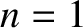tearing mode that reconnects magnetic flux principally at the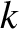th rational surface in our example tokamak plasma. Let us now suppose that the amount of reconnected magnetic flux is sufficiently large that the plasma response at this surface lies in the nonlinear regime, which is equivalent to supposing that the magnetic island width at theth rational surface is much greater than the linear layer width. (See Section 5.16.) Given that the mode is assumed not to interact strongly with the other rational surfaces in the plasma, due to the assumed strong shielding present at these surfaces, the analysis of Chapter 12 implies that the frequency of the mode can be written [10,18]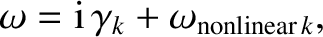(14.150)

where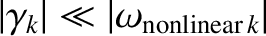, and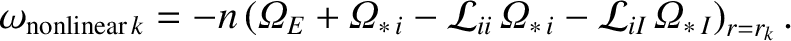(14.151)

Here, the impurity ion diamagnetic frequency profile,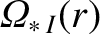, is defined in Equation (A.78). Moreover, the dimensionless neoclassical quantities,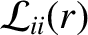and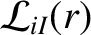, are defined in Equation (A.80). The previous two equations are a generalization of Equation (12.11) that takes the presence of impurity ions in the plasma into account, does not assume a small fraction of trapped particles, and does not assume that the plasma at theth rational surface is in the banana collisionality regime [11,14,16]. The previous two equations imply that a nonlinear tearing mode is entrained by the ion fluid at theth rational surface. It follows from Equation (14.130) that the normalized mode rotation frequency at the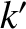th rational surface, where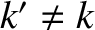, is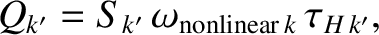(14.152)

where the nonlinear natural frequencies,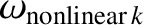, are specified in Table 14.5. Here, we have again neglected the comparatively small growth-rate of the mode with respect to its comparatively large real frequency in the laboratory frame. Note that the nonlinear natural frequencies are all significantly different from one another, and are also significantly different from the linear natural frequencies.

Table: 14.7 Nonlineartearing stability indices in KSTAR discharge #18594 at time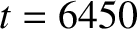ms.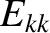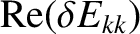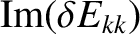1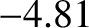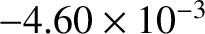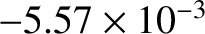2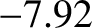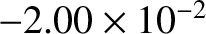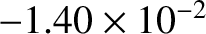3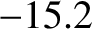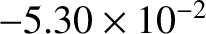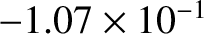4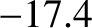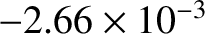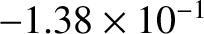If we repeat the calculation made in the previous section, replacing the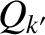values specified in Equation (14.143) with those specified in Equation (14.152), then we obtain the results shown in Table 14.7. As before, it can be seen that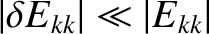for all possible nonlineartearing modes. Thus, the fact that nonlinear tearing modes effectively corotate with the ion fluid, rather than the electron fluid, does not change the conclusion of the previous section that there is sufficient rotational shear present in the plasma to ensure that a nonlineartearing mode can only reconnect magnetic flux at one rational surface at a time.

Thus, we have effectively answered the question posed at the end of Section 14.13. The full shielding response model most accurately describes a tearing mode in a realistic tokamak plasma. Note that plasmas in tokamak fusion reactor reactors are likely to possess significantly less rotation shear than KSTAR plasmas (because they will not rotate as fast as KSTAR plasmas—see Section 5.15), but much larger Lundquist numbers. The two effects largely offset one another. Hence, we conclude that the full shielding response model is also likely to apply to tokamak fusion reactors.